# Logarithmic scoring rule

## Definition

The logarithmic scoring rule is a scoring rule used to measure how well a given assignment of probabilities to values of a random variable performs on some real-world instances of the random variable. Explicitly, consider a random variable$X$ that can take$n$ distinct values$1,2,\dots,n$. Suppose we estimate probabilities$p_1,p_2,\dots,p_n$ for these values respectively (with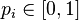$p_i \in [0,1]$,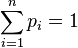$\sum_{i=1}^n p_i = 1$). The logarithmic scoring rule works as follows: for every instance of the random variable$X$, we assign score equal to the negative of the logarithm of the corresponding probability$p_i$. Explicitly, if the instances are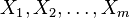$X_1,X_2,\dots,X_m$, the total score is: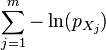$\sum_{j=1}^m -\ln(p_{X_j})$

Note that the base of the logarithms does not matter: it could be any fixed number greater than 1.

The smaller the value of the score with the logarithmic scoring rule, the better the assignment of probabilities has performed according to the rule.

## Facts

• Logarithmic scoring rule is proper: In expectation, if the actual probabilities of the values are$q_1,q_2,\dots,q_n$ respectively, then the choice of values that minimizes our expected score is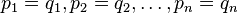$p_1 = q_1, p_2 = q_2, \dots, p_n = q_n$.

## Relation with logarithmic loss functions in logistic regression

The logarithmic loss function used in logistic regression relies on the logarithmic scoring rule, but with the following twist: the probabilities are not fixed numbers, but themselves depend on parameters that vary with the random instances that we are presented.